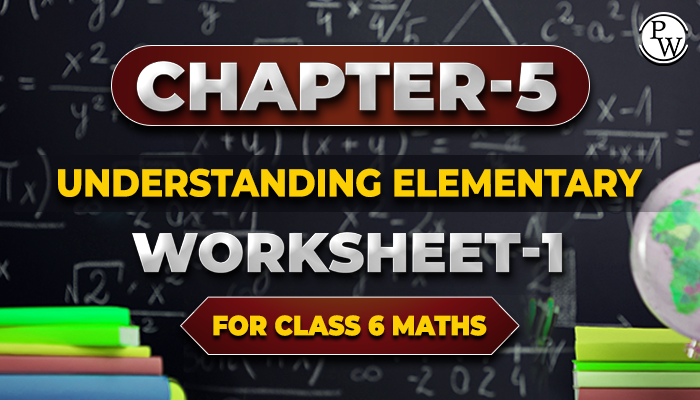# chapter-5 Understanding Elementary Worksheet-1 for class 6 maths

## Different methods of comparison will be studied in this section:

• Comparison by observation
• Comparison by tracing
• Comparison using a ruler and a divider
• The distance between the last points of a part of a line is its length.
• A description of the various tools for measuring length is provided.
• Graduated rulers and dividers help compare the size of line segments.### Questions based on Angles which will be discussed in this chapter are:

• Right angle
• Straight angle
• Complete angle
• Acute angle
• Obtuse angle
• Reflex angle

Angles are defined by taking the example of clocks, directions, stairs, and various other real-life examples. While taking a clock example, the revolution and right angles are connected.

The concept of measuring angles will also be explained in this chapter. The angle is measured in degrees. The angle used to measure the degree is the protractor. One has to understand how a protractor is used and its different features, such as scales, straight edges, etc.

### Other topics the chapter focuses on:

Perpendicular lines and perpendicular bisector: Two horizontal lines are perpendicular when the angle between them is 90 °.

Classification of Triangles: Triangles can be divided based on their angles and the length of their sides.

1. A triangle with three unequal sides is called the Scalene Triangle.
2. A triangle with two equal sides is called the Isosceles Triangle.
3. A triangle having three equal sides is called the Equilateral Triangle.
4. If each angle is less than 90 °, the triangle is called an acute-angled triangle.
5. If one angle is the right angle, a triangle is called a right triangle.
6. If anyone's angle is greater than 90 °, then the triangle is called the obtuse-angled triangle.

Quadrilate​rals: Types of quadrilaterals are discussed in this chapter:

1. Rectangle
2. Square
3. Parallelogram
4. Rhombus
5. Trapezium

Three-dimensional shapes:

1. Prism
2. Pyramid
3. Sphere
4. Cone
5. Cylinder
6. Cube and cuboid

Academic team of Physics Wallah prepared worksheet and questions for chapter Understanding Elementary Shapes for all students who are in class 6. Before solving the worksheet of Physics Wallah it is highly recommend reading the theory of chapter and solve the questions given in your textbook . Do follow NCERT Solutions for class 6 Maths prepared by Physics Wallah. If any students need to take the online test to check their concepts or undertstanding then they can visit Maths Quiz for class 6.

For additional information related to the subject you can check the Maths Formula section.

Below link consist of class 6 maths chapter-5 Understanding Elementary Shapes download your free pdf copy from the below mentioned link of chapter Understanding Elementary Shapes .